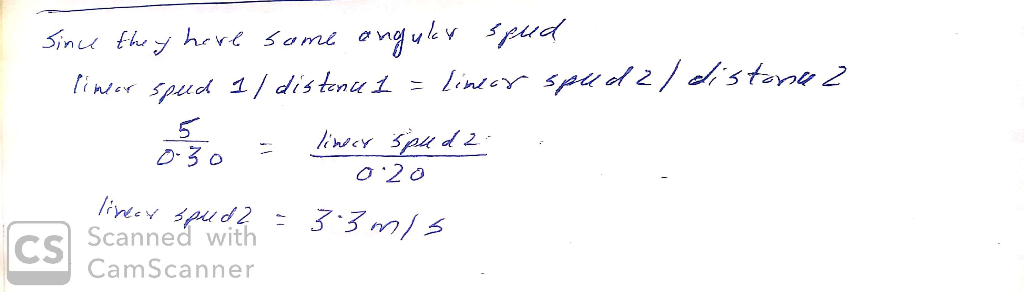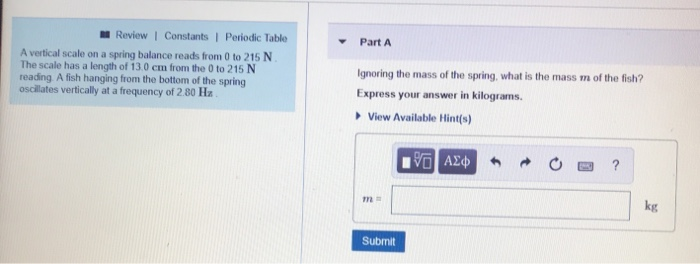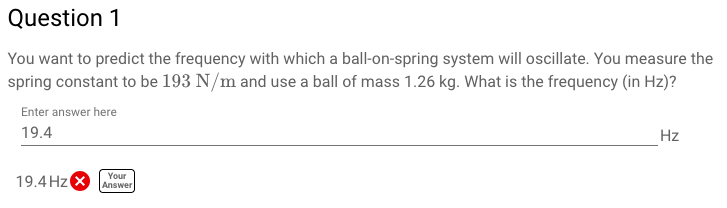# The scale of a spring balance that reads from 0 to 20.9 kg is 20.7 cm long. A package suspended from the balance is found to oscillate vertically with a frequency of 1.60 Hz. (a) What is the spring co...

The scale of a spring balance that reads from 0 to 20.9 kg is 20.7 cm long. A package suspended from the balance is found to oscillate vertically with a frequency of 1.60 Hz. (a) What is the spring constant? (b) How much does the package weigh?##### Add Answer of: The scale of a spring balance that reads from 0 to 20.9 kg is 20.7 cm long. A package suspended from the balance is found to oscillate vertically with a frequency of 1.60 Hz. (a) What is the spring co...
Similar Homework Help Questions
• ### Simple Harmonic Motion

The scale of a spring balance that reads from 0 to 17.6 kg is 22.4 cm long. A package suspended from the balance is found to oscillate vertically with a frequency of2.36 Hz. (a) What is the spring constant? (b) How much does the package weigh?

• ### A vertical scale on a spring balance reads from 0 to 250 N . The scale has a length of 10.5 cm from the 0 to 250 N readi...

A vertical scale on a spring balance reads from 0 to 250 N . The scale has a length of 10.5 cm from the 0 to 250 N reading. A fish hanging from the bottom of the spring oscillates vertically at a frequency of 2.75 Hz . Ignoring the mass of the spring, what is the mass m of the fish?

• ### help me out . i will rate lifesavor . asap. thanks thanksChapter 15, Problem 85The scale of a spring balance that reads from 0 to 46.1 kg is16.9 cm long. A package suspended from the balance is found tooscillate vertically with a frequency of1.70 Hz.(a) What is the spring constant?(b) How much does the package weigh?

• ### A 50-cm-long spring is suspended from the ceiling. A 290 g mass is connected to the...

A 50-cm-long spring is suspended from the ceiling. A 290 g mass is connected to the end and held at rest with the spring unstretched. The mass is released and falls, stretching the spring by 26 cm before coming to rest at its lowest point. It then continues to oscillate vertically. What is the spring constant? What is the amplitude of the oscillation?

• ### Review | Constants| Periodic Table ▼ Part A A vertical scale on a spring balance reads...Review | Constants| Periodic Table ▼ Part A A vertical scale on a spring balance reads from 0 to 215 N The scale has a length of 13.0 cm from the 0 to 215 N reading A fish hanging from the bottom of the spring oscillates vertically at a frequency of 2.80 Ha ignoring the mass of the spring, what is the mass m of the fish? Express your answer in kilograms. View Available Hint(s) kg Submit

• ### A spring with spring constant is suspended vertically from a support and a mass is attached. The...

A spring with spring constant is suspended vertically from a support and a mass is attached. The mass is held at the point where the spring is not stretched. Then themass is released and begins to oscillate. The lowest point in the oscillation is 46.0cm below the point where the mass was released. What is the oscillation frequency?

• ### Question 1 You want to predict the frequency with which a ball-on-spring system will oscillate. You...Question 1 You want to predict the frequency with which a ball-on-spring system will oscillate. You measure the spring constant to be 193 N/m and use a ball of mass 1.26 kg. What is the frequency (in Hz)? Enter answer here 19.4 Hz Your Answer

• ### A 10-cm-long spring is attached to theceiling. When a2.0 kg mass is hung from it,the spring stretches to a length of 15...

A 10-cm-long spring is attached to theceiling. When a2.0 kg mass is hung from it,the spring stretches to a length of 15 cm. a.What is the spring constant k? b.How long is the spring when a 3.0kg mass is suspended from it?

• ### A 10-cm-long spring is attached to theceiling. When a2.0 kg mass is hung from it,the spring stretches to a length of 15...

A 10-cm-long spring is attached to theceiling. When a2.0 kg mass is hung from it,the spring stretches to a length of 15 cm. a.What is the spring constant k? b.How long is the spring when a 3.0kg mass is suspended from it?

• ### A spring stretches 25.7 cm from equilibrium when it hangs vertically with a 1.56 kg mass...

A spring stretches 25.7 cm from equilibrium when it hangs vertically with a 1.56 kg mass attached to the spring. a) What is the spring constant of the spring? b) If the spring is pulled further down and released, what is the frequency of the oscillation?

Need Online Homework Help?